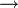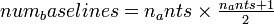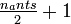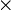# Windowed X-Engine¶

Block: Windowed X-Engine (`win_x_engine`)
Block Author: Jason Manley, Aaron Parsons, Terry Filiba
Document Author: Jason Manley

## Summary¶

CASPER X engine with added internal valid data masking functionality. Based on Aaron Parsons’ design.

Parameter Variable Description
Number of antennas n_ants Number of antennas to process.
Bit width of samples in n_bits Bit width of each input sample number. Usually set to 4, resulting in 16 bit input numbers (2 polarizations, complex numbers).
Accumulation length acc_len Specified per antenna.
Multiplier latency mult_latency Used to set the latency of internal multipliers.
BRAM latency bram_latency Used to set the latency of internal BRAMs.
Implementation: Multiplier type use_ded_mult Select the type of multipliers to use. Can be a single number or array - see below.
Implementation: Delay type use_bram_delay Selects the type of delays to implement. Single number configures all internal taps.

## Ports¶

Port Dir Data Type Description
ant in variable width. see below. Input port for incoming antenna data.
sync_in in boolean Synchronization pulse. New window begins clock cycle after sync received.
window_valid in boolean Indicates incoming antenna data is valid. Must remain constant for acc_len*n_ants.
acc out variable width. see below. Output data.
valid out boolean Indicates data on acc is valid.
sync_out out boolean Passthrough for sync pulses.

## Description¶

### Introduction¶

The CASPER X engine is a streaming architecture block where complex antenna data is input and accumulated products (for all cross-multiplications) are output in conjugated form. Because it is streaming with valid data expected on every clock cycle, data is logically divided into windows. These windows can either be valid (in which case the computation yields valid, outputted results) or invalid (in which case computation still occurs, but the results are ignored and not presented to the user).

### Input format¶

Data is input serially: `antenna A, antenna B, antenna C` etc. Each antenna’s data consists of dual polarization, complex data. The bit width of each component number can be set as a parameter, `n_bits`. The X-engine thus expects these four numbers of `n_bits` to be concatenated into a single, unsigned number. CASPER convention dictates that complex numbers are represented with higher bits as real and lower bits as imaginary. The top half of the input number is polarization one and the lower half polarization two.

The internals of the block are reset with the reception of a sync pulse. A new window begins on the very next clock cycle. Each window is `int_len` * `n_ants` clock cycles long. The data for each antenna is input for `acc_len` clock cycles.

For example, for `n_bits` of 4 and `acc_len` of 2, the input to the X-engine would be 16 bits every clock cycle mapped as follows:

 … t4 t3 t2 t1 t0$rightarrow$ … C1real B1real B1real A1real A1real most_sig 4b$rightarrow$ … C1imag B1imag B1imag A1imag A1imag 4b$rightarrow$ … C2real B2real B2real A2real A2real 4b$rightarrow$ … C2imag B2imag B2imag A2imag A2imag least_sig 4b$rightarrow$

X-engine input with `acc_len` of 2.

The `window_valid` line is expected to remain constant for the duration of each window. If it is high, the output is considered valid and captured into the output FIFO buffer. With the close of that window, the output will be presented to the user as valid data on every second clock pulse. If `window_valid` was held low, the data is ignored.

With the close of one window, anther begins directly afterwards. Data can thus be streamed in and out continuously, while a sync pulse will force the start of a new window.

### Output Format¶

The windowed X-engine will produce$num_baselines1$ valid outputs. The unwindowed x engine produces$num_baselines2$ results. The extra valids are a result of the algorithm employed and are masked out by the internal `x_engine_mask`.

Generally, the output of the X-engine configured for `N` antennas can be mapped into a table with$tfrac$ columns and N rows as follows:

 1st 0$times$ 0 0$times$ N 0$times$ (N-1) 0$times$ (N-2) …$rightarrow$ 2nd 1$times$ 1 0$times$ 1 1$times$ N 1$times$ (N-1) …$rightarrow$ 3rd 2$times$ 2 1$times$ 2 0$times$ 2 2$times$ N …$rightarrow$ 4th 3$times$ 3 2$times$ 3 1$times$ 3 0$times$ 3 …$rightarrow$ 5th 4$times$ 4 3$times$ 4 2$times$ 4 1$times$ 4 …$rightarrow$ 6th 5$times$ 5 4$times$ 5 3$times$ 5 2$times$ 5 …$rightarrow$ … … … … … …$rightarrow$

Each table entry represents a valid output. Data is read out right to left, top to bottom. Bracketed values are from previous window.

As an example, consider the output for a 4 antenna system (with antennas numbered A through D):

 1st AA prev win DA prev win CA 2nd BB AB prev win BD 3rd CC BC AC 4th next win AA CD BD 5th next win BB next win AB DB

Boldfaced type represents current valid window of data. Data is read out right to left, top to bottom. Non-boldfaced data is masked.

Thanks to the inclusion of the `x_engine_mask` block, X-engine output duplicates (observed in rows 5 and 6 above) are automatically removed. The output of a 4 antenna windowed X-engine is thus `AA, AB, BB, AC, BC, CC, BD, CD, DD, DA`.